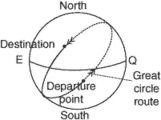geodetic line

Also found in: Dictionary, Thesaurus, Wikipedia.

geodetic line

[¦jē·ə¦ded·ik ′līn]
(geodesy)
The shortest line between any two points on the surface of the spheroid.

geodesicGreat circle is the shortest distance between two points.
The shortest distance between two points on any surface. The shortest distance between two places on earth derived mathematically is called a geodetic line.

geodesic line

The shortest line, on a mathematically derived surface, between two points. Also called geodesic. A geodesic line on the spheroidal earth is called a geodetic line. It is a portion of the great-circle arc. See geodesic.
Site: Follow: Share:
Open / Close